# When Is An Equation Linear? (5 Key Things You Should Know)

Linear equations are used in math throughout algebra and beyond.  Knowing how to solve a linear equation helps us to solve other types of equations, including quadratics, cubics, and other higher-order equations.

So, when is an equation linear?  A linear equation in 1 variable has the form ax + b = 0. Often, the coefficients a and b are real numbers. The only exponents for the variables in a linear equation are 0 (for the constant term b) and 1 (for the linear term ax). There are no radicals or negative exponents in a linear equation.

Of course, a more general form of a linear equation is a1x1 + a2x2 + … + anxn + b = 0, where each xi is a different variable.  In this case, it is still true that the only exponent for any variable is either 0 (for the constant term b) or 1 (for the linear terms aixi).

In this article, we will focus on linear equations in 1 variable, of the form ax + b = 0.  We will answer some common questions about linear equations, and we will look at some examples to make the concept clear.

Let’s get started.

## When Is An Equation Linear?

A linear equation has the following form:

• a1x1 + a2x2 + … + anxn + b = 0

where x1, x2, … xn are n separate variables, with coefficients a1, a2, … , an and a constant term b.  Usually, a1, a2, … , an and b are real numbers (but they could also be complex, rational, integers, etc.).

### Linear Equation In One Variable

More specifically, a linear equation in one variable has the following form:

When Does A Quadratic Have No Solut...
When Does A Quadratic Have No Solution?
• ax + b = 0

where a is the linear coefficient and b is the constant coefficient.

### Linear Equation In Two Variables

We can also look at a linear equation in two variables, which has the form:

• ax + by + c = 0

where a and b are linear coefficients and c is the constant coefficient.

### Can Linear Equations Have Exponents?

A linear equation can only have exponents of 0 and 1 for its variables.  There are no exponents of 2, 3, or higher.

#### Example 1: A Linear Equation With Exponents

For example, the linear equation 2x + 6 = 0 has:

• an exponent of 0 for the constant term, since 6 = 6*1 = 6*x0
• an exponent of 1 for the linear term, since 2x = 2x1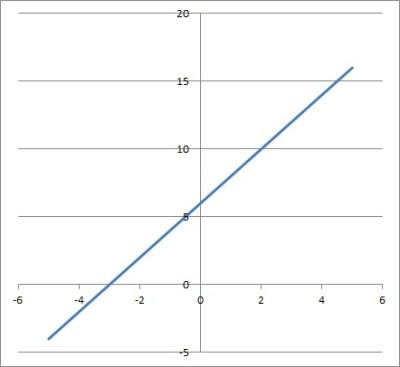This is the graph of the linear equation y = 2x + 6.

#### Example 2: A Nonlinear Equation With Exponents

An exponent of 2 in an equation would suggest a polynomial of degree 2 (such as a quadratic equation of the form ax2 + bx + c = 0).

For example, the equation x2 – 9 = 0 is not a linear equation, due to the presence of the quadratic term x2 (which has an exponent of 2). You can see the graph of this quadratic equation below (which is clearly not a line).

#### Example 3: A Nonlinear Equation With Exponents

An exponent of 3 in an equation would suggest a polynomial of degree 3 (such as a cubic equation of the form ax3 + bx2 + cx + d = 0).

For example, the equation x3 – 125 = 0 is not a linear equation, due to the presence of the cubic term x3 (which has an exponent of 3). You can see the graph of this cubic equation below (which is clearly not a line).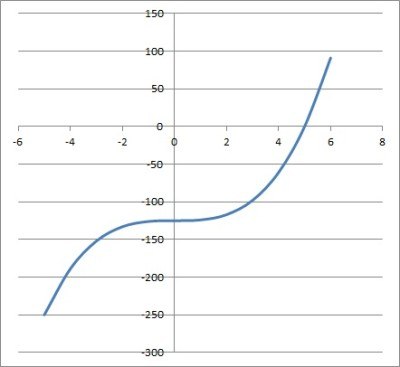This is the graph of the cubic equation y = x3 – 125.

### Can Linear Equations Have Square Roots?

A linear equation can have a square root in a coefficient. However, a linear equation cannot have a variable under a square root (or a variable under any other radicals for that matter).

Remember that a linear equation can only have exponents of 0 and 1 for its variables.

A radical of the form n√xm is equivalent to xm/n, but there are no exponents of 1/2 (for a square root), 1/3 (for a cube root), or any other fractional values in a linear equation.

#### Example 1: A Linear Equation With A Radical (Square Root)

For example, the equation 4x + √3 = 0 is a linear equation.  The only powers of x are 0 (for the constant term √3) and 1 (for the linear term 4x)

You can see the graph of y = 4x + √3 below.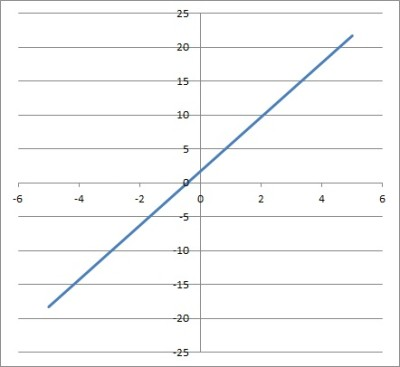This is the graph of the linear equation y = 4x + √3.

#### Example 2: A Nonlinear Equation With A Radical (Square Root)

For example, the equation √x + 3 = 0 is not a linear equation.  We can rewrite √x as x1/2, which means the equation is x1/2 + 3 = 0 (you can see the graph of y = √x + 3 below).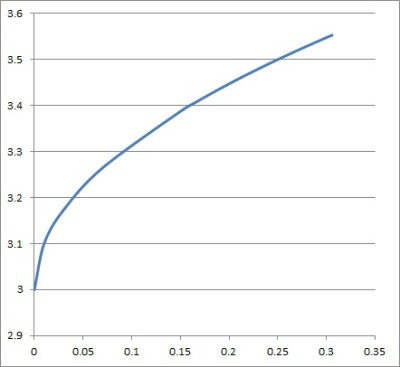This is the graph of the nonlinear equation y = √x + 3.

Since this equation has an exponent of 1/2 (which is neither 0 nor 1), the equation is not linear.  Also, the equation does not have a linear term (such as 4x), so it cannot be linear.

#### Example 3: A Nonlinear Equation With A Radical (Cube Root)

For example, the equation 3√x – 4 = 0 is not a linear equation.  We can rewrite 3√x as x1/3, which means the equation is x1/3 – 4 = 0 (you can see the graph of y = 3√x – 4 below).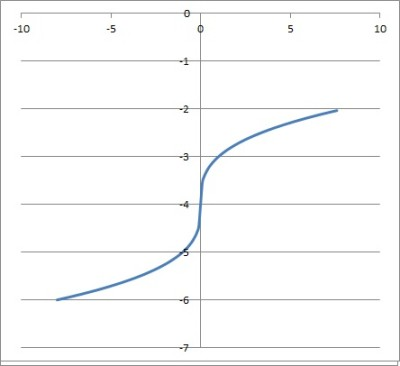The graph of the nonlinear equation y = 3√x – 4.

Since this equation has an exponent of 1/3 (which is neither 0 nor 1), the equation is not linear.  Also, the equation does not have a linear term (such as 4x), so it cannot be linear.

### Can Linear Equations Have Negative Numbers?

A linear equation can have negative numbers for the linear and/or constant coefficients.  However, a linear equation cannot have a negative number as an exponent for any variable.

#### Example 1: A Linear Equation With Negative Numbers (Coefficients)

For example, the equation -4x – 8 = 0 is a linear equation, since the variable x only has powers of 0 (the constant term -8) and 1 (the linear term -4x).

You can see the graph of y = -4x – 8 below.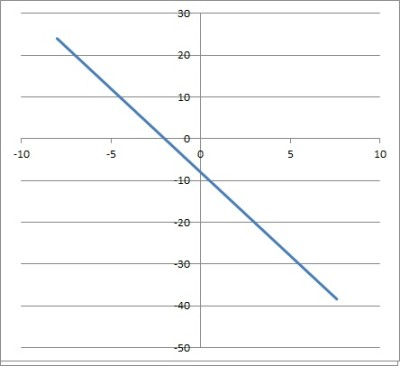This is the graph of the linear equation y = -4x – 8.

#### Example 2: A Nonlinear Equation With Negative Exponents (Negative Powers)

For example, the equation x-1 – 4 = 0 is not a linear equation, since it has a negative exponent for the variable x (you can see the graph of y = x-1 – 4 below).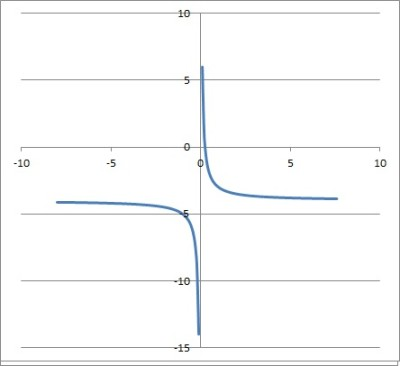The graph of the nonlinear equation y = x-1 – 4.

Since this equation has an exponent of -1 (which is neither 0 nor 1), the equation is not linear.  Also, the equation does not have a linear term (such as 4x), so it cannot be linear.

### Can Linear Equations Have Fractions?

A linear equation can have fractions for coefficients, but there cannot be fractions in the exponent of a variable.

#### Example 1: A Linear Equation With Fractions (Coefficients)

For example, the equation 3x – 1/2 = 0 is a linear equation, since the variable x only has powers of 0 (the constant term -1/2) and 1 (the linear term 3x).

You can see the graph of y = -3x – 1/2 below.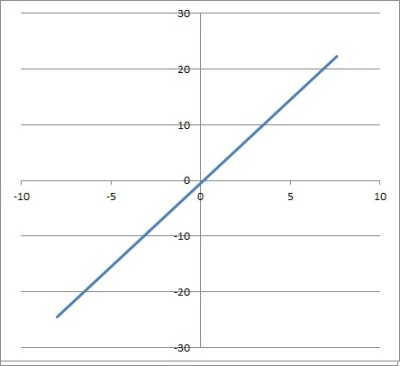The graph of the linear equation y = 3x – 1/2.

#### Example 2: A Nonlinear Equation With Fractional Exponents (Powers)

For example, the equation x2/3 – 64 = 0 is not a linear equation, since it has a fractional exponent for the variable x (you can see the graph of y = x2/3 – 64 below).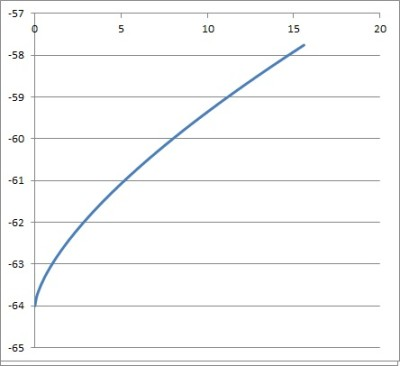This is the graph of the nonlinear equation y = x2/3 – 64.

Since this equation has an exponent of 2/3 (which is neither 0 nor 1), the equation is not linear.  Also, the equation does not have a linear term (such as 4x), so it cannot be linear.

### Can Linear Equations Have Decimals?

A linear equation can have decimals for coefficients, but there cannot be decimals in the exponent of a variable.

#### Example 1: A Linear Equation With Decimals (Coefficients)

For example, the equation 0.2x + 0.8 = 0 is a linear equation, since the variable x only has powers of 0 (the constant term 0.8) and 1 (the linear term 0.2x).

You can see the graph of y = 0.2x + 0.8 below.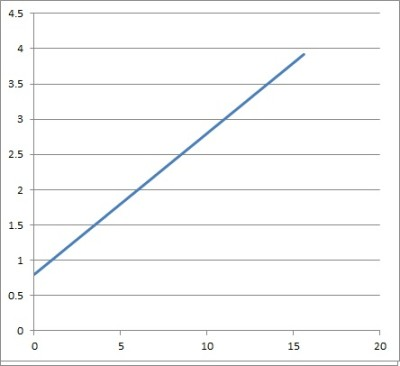The graph of the linear equation y = 0.2x + 0.8.

#### Example 2: A Nonlinear Equation With A Decimal Exponent (Powers)

For example, the equation x0.6 – 8 = 0 is not a linear equation, since it has a fractional exponent for the variable x (you can see the graph of y = x0.6 – 8 below).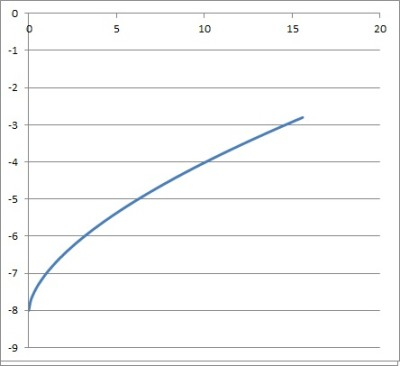The graph of the nonlinear equation y = x0.6 – 8.

Since this equation has an exponent of 0.6 (which is neither 0 nor 1), the equation is not linear.  Also, the equation does not have a linear term (such as 4x), so it cannot be linear.

### Can Linear Equations Have No Solution?

A linear equation can have no solution if the values of the variables and/or coefficients are restricted.

#### Example 1: A Linear Equation With No Solution

Consider the linear equation ix + 1 = 0, where x must be a real number.  When we try to solve, we get:

• ix + 1 = 0
• ix = -1
• x = -1/i
• x = i

We know that i is an imaginary number, but x must be real.  Thus, there is no solution to this equation.

Note: if we allow complex (imaginary) numbers for x, then x = i would be a solution.

#### Example 2: A Linear Equation With No Solution

Consider the linear equation 3x + 2 = 0, where x must be an integer.  When we try to solve, we get:

• 3x + 2 = 0
• 3x = -2
• x = -2/3

We know that -2/3 is a rational number, but x must be an integer.  Thus, there is no solution to this equation.

Note: if we allow rational numbers for x, then x = -2/3 would be a solution.

### Can Linear Equations Have More Than 2 Variables?

A linear equation can have more than 2 variables.  In fact, a linear equation can have n variables, where n is any whole number greater than 0.

We are already familiar with the general form for some of these linear equations:

• 1 variable: ax + b = 0 [the solution is just a single number]
• 2 variables: ax + by + c = 0 [the solution is an entire line in a 2D coordinate plane]

For more variables, we just add another term with a new variable:

• 3 variables: ax + by + cz + d = 0 [the solution is an entire plane in a 3D space]
• n variables: a1x1 + a2x2 + … + anxn + b = 0

## Conclusion

Now you know what makes an equation linear, and what is not allowed in these equations.  You also have some examples and the answers to some common questions about linear equations.

You can learn about the difference between expressions and equations here.

I hope you found this article helpful.  If so, please share it with someone who can use the information.

~Jonathon